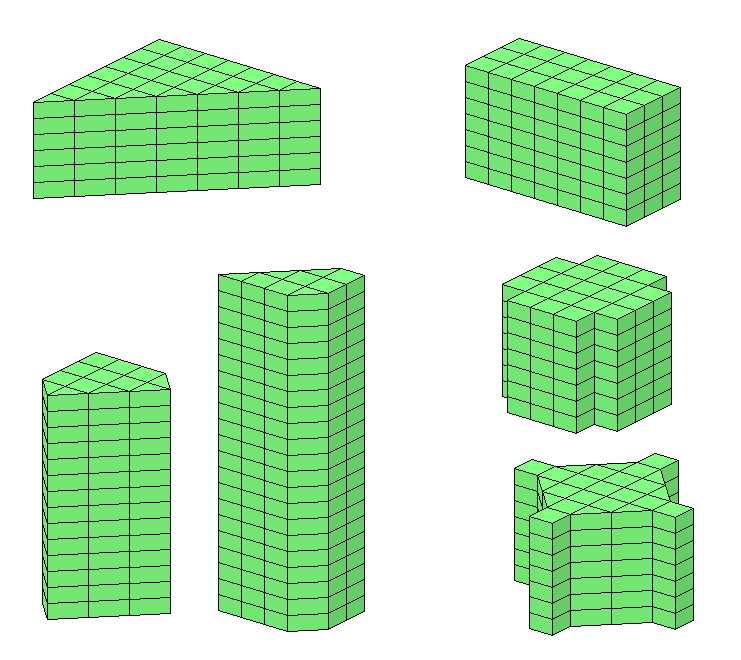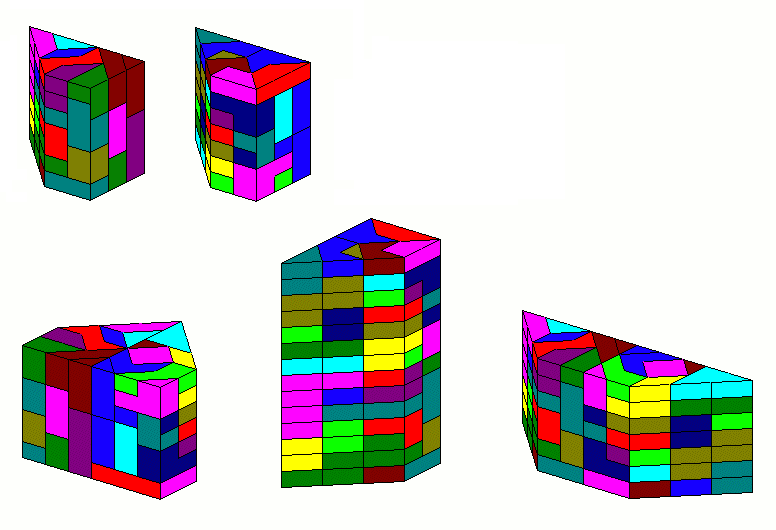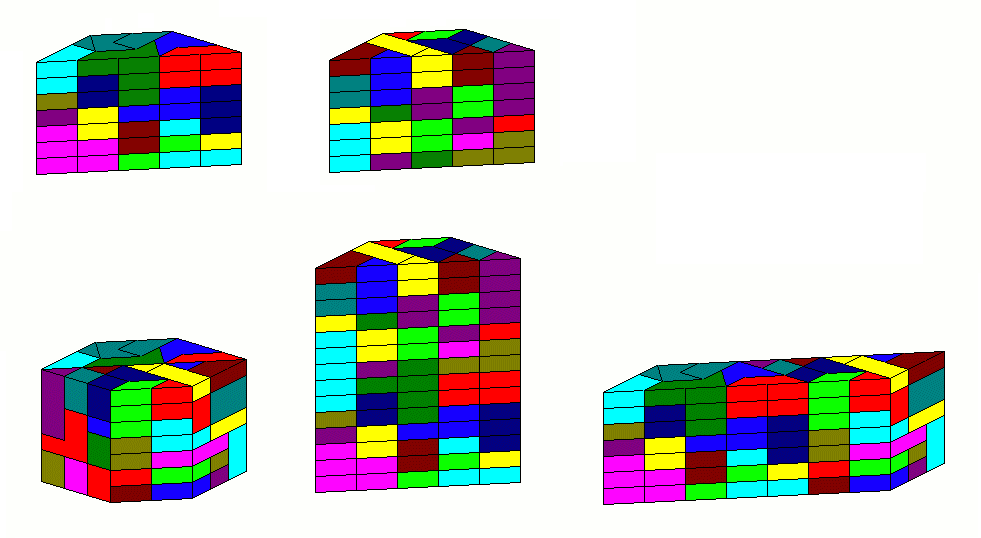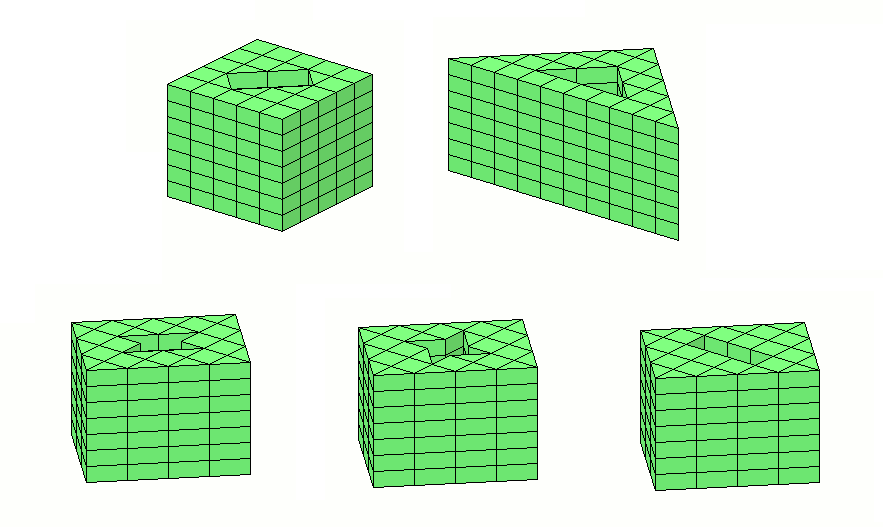## Combined Set of Stacked Tritans and Tetratans

There are no constraints for constructions with this set and the total number of triangles 66*4+10*3=264+30=294 can be well factorized, because 294=2*3*7*7.

Therefore posssible heights for prisms are 6, 7, 14 and 21 with cross-sections consisting of 49, 42, 21 or 14 tans, respectively.With height=6 a perfect replica of a tan can be made. The layers for this prism are here.

For height=7 on the right side of the picture we can get a 3*7*7 box and prisms with rotational symmetry like the cross tower or the castle. To get solutions for these figures I split the whole prism into two parts, because the computer program is more efficient on small areas.

The prism with height=14 has a symmetric pentagon as cross-section whereas the cross-section of the prism with height=21 is irregluar.

Two congruent rectangular trapeziums of height=7 can be used to make prisms with a pentagon or trapeziums as cross-section. The layers for the two prism are here.Two congruent isosceles trapeziums of height=7 can be used to make prisms with a hexagon, a trapezium or a parallelogram as cross-section. The layers for the two prism are here.If we allow holes in the cross-section, a square with a rectangular hole or a triangle with a triangle hole are possible symmetric bottom areas. Starting with a 3h*4h rectangle we can leave out a hexatan hole to get an area of 42 tans for the cross-section. Some examples are shown.Here are the layers for the first two figures and for the prisms with hexatan holes.

Back
Home